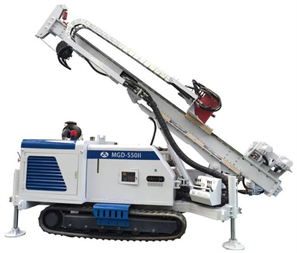#### 期刊菜单

Study on the Technology Method of Vibration Sinking Casing

Abstract: Mud wall protection is often used in loose, soft and collapsible strata, which is easy to cause environmental pollution. However, there are some problems such as insufficient depth of anhydrous static pressure sinking into the casing. This paper studies the process method of sinking the casing with acoustic vibration, introduces the process flow and construction steps of acoustic vibration sinking, and carries out the field test of acoustic vibration sinking. The test shows that the speed of acoustic vibration sinking is the fastest in miscellaneous fill, the second in soft plastic clay layer and the slowest in fine sand layer. By calculating the end resistance and side resistance of the casing, the attenuation value of the drilling rig vibration force at the bottom of the casing is obtained, and then the theoretical limit depth of sinking into the casing is calculated. This process method has the characteristics of fast pipe sinking speed, simple construction process and no mud pollution. It can solve the wall protection problem of complex strata, ensure the construction progress and quality of drilling engineering, and lay a foundation for application projects such as soft soil sampling, environmental pollution site investigation and placer gold exploration.

1. 前言

2. 声频振动钻机介绍

3. 声频振动沉管工艺优势Figure 1. MGD-S50 II acoustic vibration drill

4. 声频振动沉管试验

4.1. 场地地层Table 1. Statistics of site strata

4.2. 声频振动沉管过程

1) 破除地表硬层

2) 取样

3) 振动沉入套管

4) 继续振动取样

5. 振动沉管速度分析Table 2. Statistics of vibration sinking velocityFigure 2. Variation curve of sinking casing velocity with casing shoe depthFigure 3. Variation curve of average velocity of sinking casing with ground layer

6. 地层阻力与振动力关系研究

$F={F}_{\text{1}}+{F}_{\text{2}}$ (1)

$F$ ——套管受到的总阻力值，kN；

${F}_{\text{1}}$ ——套管端阻力，kN；

${F}_{\text{2}}$ ——套管侧摩阻力，kN。

${F}_{\text{1}}={q}_{pk}×{S}_{1}$ (2)

${q}_{pk}$ ——极限端阻力标准值，kPa；

${S}_{1}$ ——套管底端面积，m2

${F}_{\text{2}}={q}_{sik}×{S}_{2}$ (3)

${q}_{sik}$ ——桩的极限侧阻力标准值，kPa；

${S}_{2}$ ——套管侧面接触地层部分总面积，m2

${q}_{sk}$ 的取值详见表3 。Table 3. Standard value of ultimate end resistance of pile q s k (kPa)Table 4. Standard value of ultimate lateral resistance of pile q s i k (kPa)

${F}_{\text{1}}=\text{2400}×\text{1}\text{.73}×{\text{10}}^{-3}=\text{4}\text{.152}\text{\hspace{0.17em}}\text{kN}$Table 5. Ultimate side friction resistance of single-layer soil

$F=135.792\text{\hspace{0.17em}}\text{kN}$

φ127 mm套管(壁厚4.5 mm)的重量是21.41 kg/m。

20根套管自重为6.423 kN。

${F}_{振动}=F-G$ (4)

G——套管自重，kN。

7. 结论

1) 地层不同分布、不同厚度，对沉入套管时需要克服的阻力计算会产生较大差别。杂填土和软塑黏土层分布较多，则所需克服阻力较小，硬塑粘土和细砂层分布较多，则所需克服阻力较大。

2) 随着钻孔深度的不断增加，套管受到的侧摩擦阻力增加值远大于套管的端阻力增加值。声频振动沉入套管工艺可以使孔壁土壤局部液化，产生间隙，能有效降低套管受到的侧摩阻力，大幅提升沉入套管的速度，满足30米目标深度的护壁要求。

3) 声频振动沉入套管工艺方法能有效解决在淤泥层、流砂层等易坍塌地层的钻探取样难题，沉管、提管速度快，无泥浆污染，场地环境清洁。该工艺方法在尾矿库勘察、浅表基质层调查、岩土工程施工、生态环境调查取样等项目中具有推广应用价值。

  周石金. 套管护壁解决松散层钻进难题[J]. 水文地质工程地质, 1999(4): 61-63.  李伟, 吴浩, 杨恺. MGD-S50Ⅱ型声频振动钻机的研制与试验[J]. 中国煤炭地质, 2017(12): 75-77+95.  黄强, 刘金砺, 高文声, 刘金波, 等. 建筑桩基技术规范. JGJ94-2008 [S]. 北京: 中国建筑工业出版社, 2008.  中华人民共和国冶金工业部. 地质钻探用钢管. YB235-70 [S]. 鞍山: 技术标准出版社, 1970.